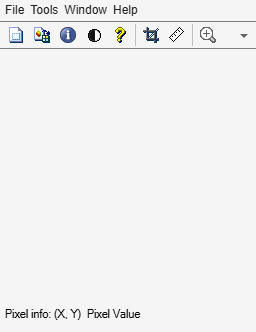# getimage

Image data from axes

## Syntax

``I = getimage(h)``
``[x,y,I] = getimage(h)``
``[___,flag] = getimage(h)``
``[___] = getimage``

## Description

````I = getimage(h)` returns the first image data contained in the graphics object `h`.```
````[x,y,I] = getimage(h)` also returns the image extent in the x and `y` direction.```
````[___,flag] = getimage(h)` also returns a flag that indicates the type of image that `h` contains.```

example

````[___] = getimage` returns information for the current axes object.```

## Examples

collapse all

Display image directly from a file using `imshow` and create a variable in the workspace that contains the image data.

`imshow rice.png``I = getimage;`

Display image directly from a file using the Image Viewer app (`imtool`) and create a variable in the workspace that contains the image data.

`h = imtool('cameraman.tif');``I = getimage(imgca);`

## Input Arguments

collapse all

Handle to a figure, axes, uipanel, or image graphics object, specified as a handle. If `h` is an axes or figure handle containing multiple images, then `getimage` uses the first image returned by `findobj(h,'Type','image')`.

## Output Arguments

collapse all

Image data, returned as a numeric array. `I` is identical to the image `CData`; it contains the same values and is of the same class as the image `CData`. If `h` is not an image or does not contain an image, then `I` is empty.

Image extent in the x direction, returned as a 2-element numeric vector of the form `[xmin xmax]`. `x` is identical to the image `XData`.

Data Types: `double`

Image extent in the y direction, returned as a 2-element numeric vector of the form `[ymin ymax]`. `y` is identical to the image `YData`.

Data Types: `double`

Image type, returned as an integer with one of these values:

Flag

Type of Image

`0`

Not an image; `I` is returned as an empty matrix

`1`

Indexed image

`2`

Intensity image with values in standard range. The standard range for `single` and `double` images is [0,1].

`3`

Intensity data, but not in standard range

`4`

RGB image

`5`

Binary image

Data Types: `double`